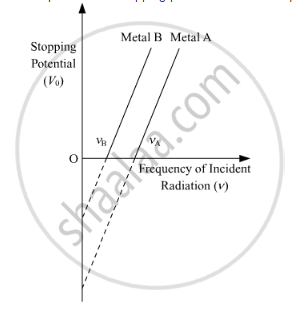# Sketch the Graphs Showing Variation of Stopping Potential with Frequency of Incident Radiations for Two Photosensitive Materials - Physics

Sketch the graphs showing variation of stopping potential with frequency of incident radiations for two photosensitive materials A and B having threshold frequencies vA > vB.

(i) In which case is the stopping potential more and why?

(ii) Does the slope of the graph depend on the nature of the material used? Explain.

#### Solution

(i)
Graph between stopping potential and the frequency of the incident radiation:From the graph, we note:

(i) The stopping potential is inversely proportional to the threshold frequency. Hence the stopping potential is higher for metal B.

(ii) The slope of the graph does not depend on the nature of the material used.

As we know,

Kmaxh− ϕ0 eV0

Dividing the whole equation by e , we get

(hv)/e-phi_0/e=V_0

From the above equation, the slope of the graph is h/e(on comparing with the straight line equation). Thus, we see that the slope is independent of the nature of the photoelectric material.

Concept: Photoelectric Effect - Hertz’S Observations
Is there an error in this question or solution?